### Homework exercise

To be solved at home before the exercise session.

1. Assume that we have an iid. random sample $$x_1, \ldots , x_{1000}$$ and we’d like to use the normal Q-Q plot to assess whether the sample came from a normal distibution. How do you expect the normal Q-Q plot to roughly look like (i.e. what general features do you expect it to have and why), if the true distribution of the data is
1. a normal distribution,
2. a right-skew distribution,
3. a left-skew distribution,
4. a bimodal distribution,
5. a distribution with light tails,
6. a distribution with heavy tails?
# i. Straight line: sample quantiles depend approximately linearly on normal quantiles

# ii. U-shaped curve: the high values are too high (long right tail) and the low values
# are too high (short left tail).

# iii. Inverse U-shaped curve: for the opposite reasons as in ii.

# iv. S-shape near the middle of the plot: the points left of median are too small and the points right of median are too large (too little mass near median)

# v. S-shape in the tails: the low values are too high (the left tail is too short) and the high values
# are too low (the right tail is too short).

# vi. Something resembling a cubic function: the low values are too low (the left tail is too long) and
# the high values are too high (the right tail is too long).

# Sample plots:
par(mfrow = c(3, 2))

x <- rnorm(1000)
qqnorm(x)
qqline(x)

x <- rexp(1000, 1)
qqnorm(x)
qqline(x)

x <- -1*rexp(1000, 1)
qqnorm(x)
qqline(x)

b <- rbinom(1000, 1, 1/2) == 1
x <- c(rnorm(1000, 3)[b], rnorm(1000, -3)[!b])
qqnorm(x)
qqline(x)

x <- runif(1000)
qqnorm(x)
qqline(x)

x <- rt(1000, 3)
qqnorm(x)
qqline(x)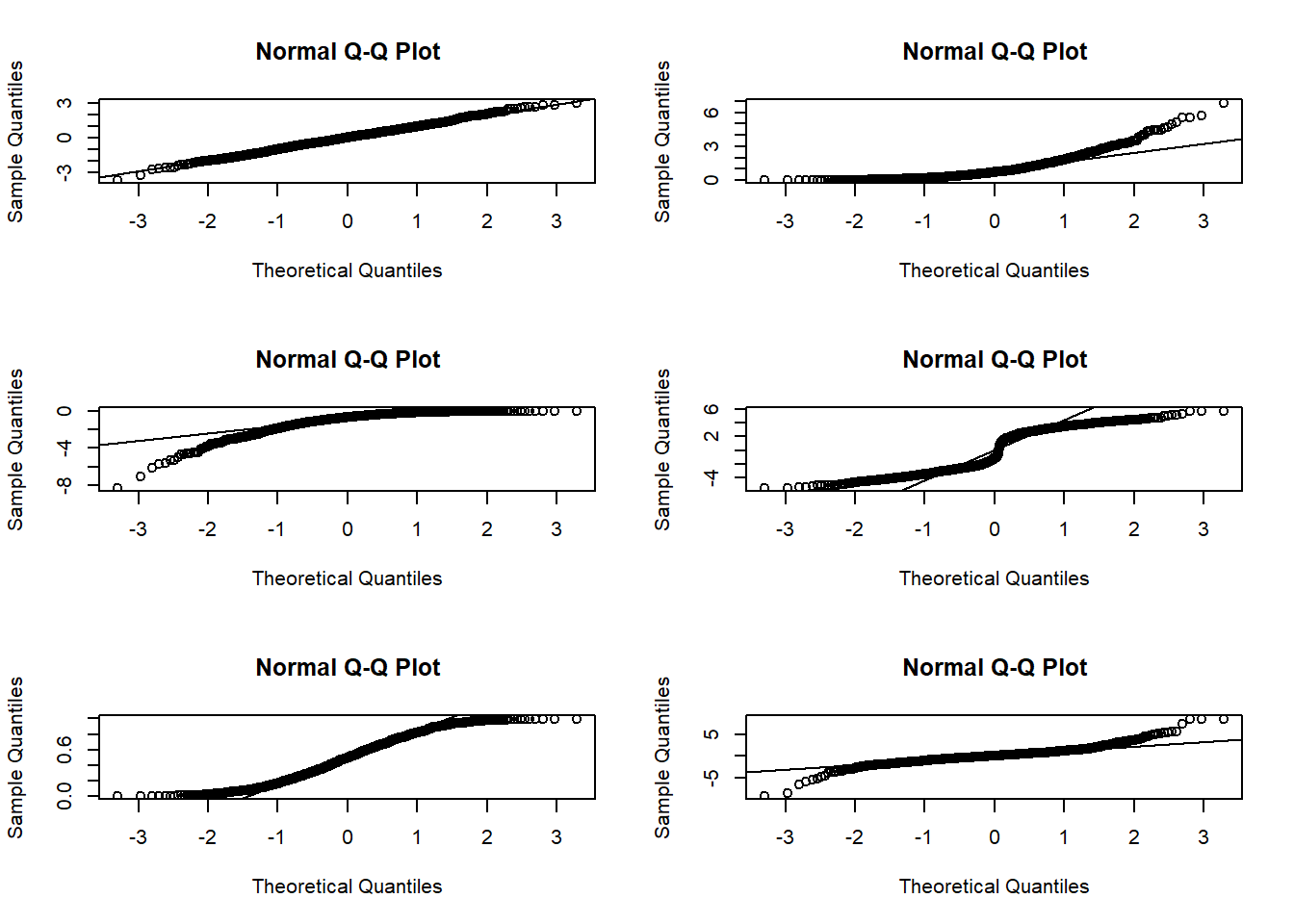par(mfrow = c(1, 1))
b. Recall the differences between the interpretations of the $\chi^2$ homogeneity test and $\chi^2$ test for independence. Come up with a practical situation where the collected data can be expressed as a 2-by-2 table and a related research question for which the correct interpretation is through
i. the $\chi^2$ homogeneity test,
ii. the $\chi^2$ test for independence.
# The key difference between the two tests is in how the data is sampled, i.e., are the margins fixed or not.

# E.g. assume we're interested in studying whether sex (female/male) has an effect on the voting preference
# (democrat/republican) in the US and for this we interview n people in the street.
# These data can be collected into a two-by-two table such that the row variable is sex and the column
# variable is voting preference.

# i. If we choose beforehands that we will interview n1 females and n2 males, then studying the independence of the two variables will be questionable (since sex is not fully random anymore with its marginal frequencies fixed). The correct interpretation is through the homogeneity test which compares two populations, in this case female and male, in their voting preferences.

# ii. If we do not choose beforehands the marginal numbers of females and males, sex is a random variable and we can measure its independence with the voting behavior. The correct interpretation is now through the test for independence.

### Class exercise

To be solved at the exercise session.

Note: all the needed data sets are either given below or available in base R.

1. The data set rock contains measurements on 48 rock samples from a petroleum reservoir. Treat the data as an iid. random sample from some distribution and test whether the distribution of shape is normal.
1. Visualize the data to obtain a preliminary idea of the possible normality of the data.
2. Use the normal Q-Q plot to gain more evidence on the normality/non-normality of the data.
3. Conduct the Bowman-Shenton (Jarque-Bera) and the Shapiro-Wilk tests of normality on significance level 0.05.
4. After all the previous, would you conclude the data to be normal (or normal enough for methods with normality assumptions)?
5. Why is the data not really iid.?
# a.
x <- rock[, 3]
# The histogram seems a bit skew but otherwise not too far from normal?
hist(x)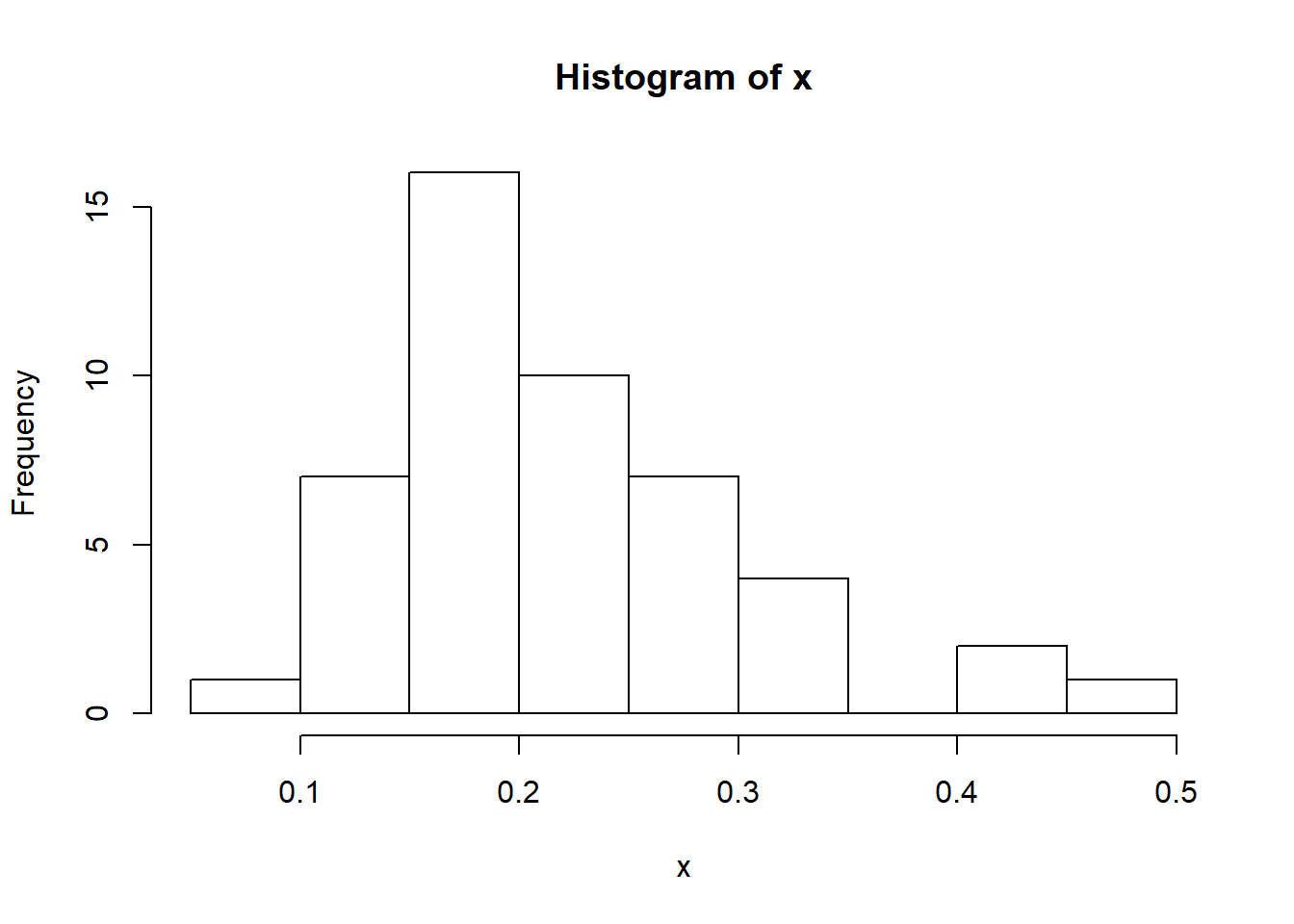# b.
# The normal Q-Q plot gives more evidence of positive skewness (it is reminiscent of part ii in Homework 1a.)
qqnorm(x)
qqline(x)

# c.
# Bowman-Shenton/Jarque-Bera
library(tseries)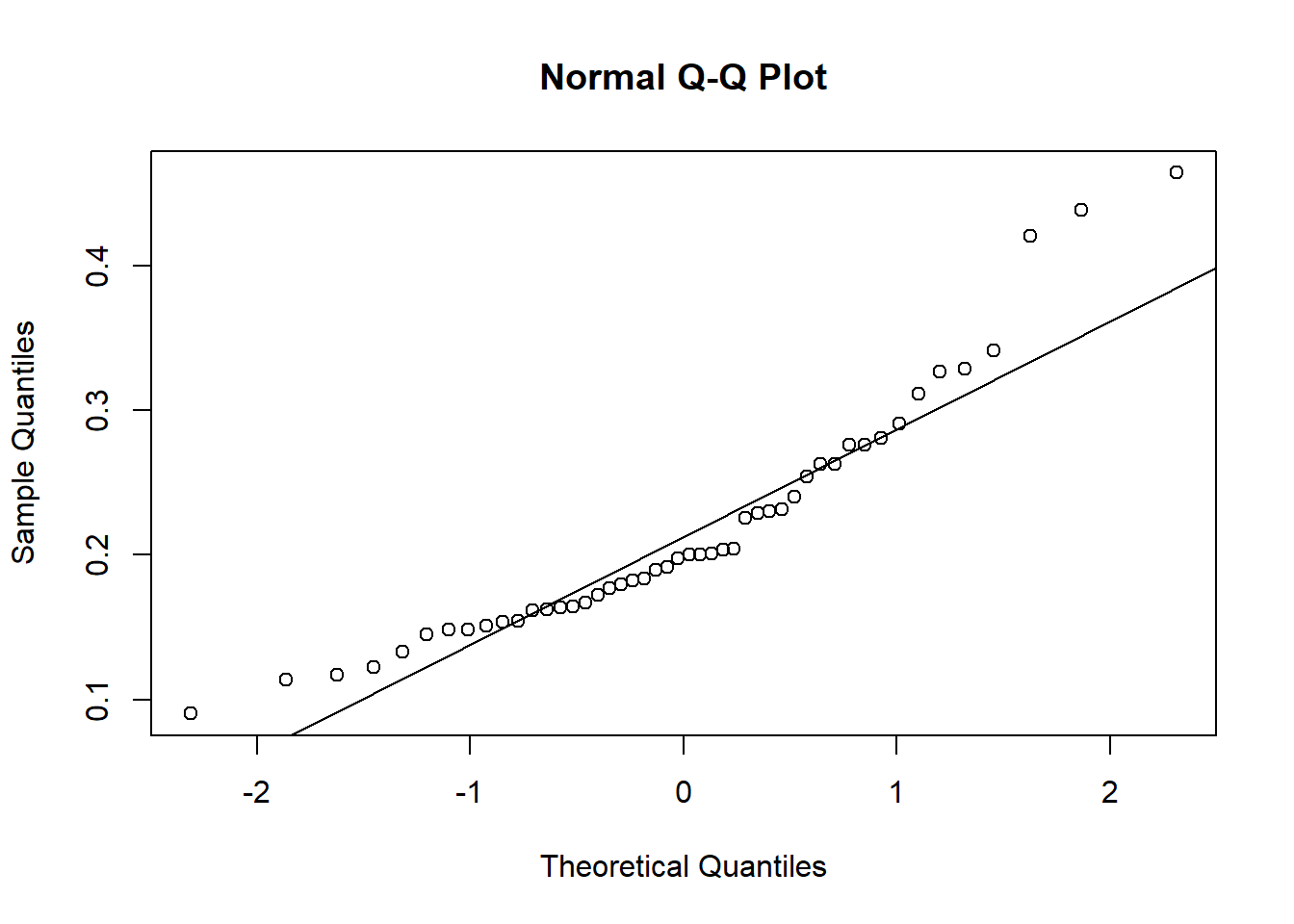jarque.bera.test(x)
##
##  Jarque Bera Test
##
## data:  x
## X-squared = 13.402, df = 2, p-value = 0.00123
# Shapiro-Wilk
shapiro.test(x)
##
##  Shapiro-Wilk normality test
##
## data:  x
## W = 0.90407, p-value = 0.0008531
# d.
# Both tests in c. reject their null hypotheses of normality. Based on all the previous evidence, the data can not be deemed normal enough to rely on normality assumptions in any further analyses.
# (Note that it is a different matter whether the next analysis steps involve methods that allow the normality assumption to be "covered" by large enough sample size (by the central limit theorem).)

# e.
# See the help file of the dataset: The sample is not iid. as, to obtain the 48 measurements, first 12 "core samples" were obtained (randomly?) and then from each of these 4 observations were taken to yield the final 48 observations. Thus, the sets of 4 observations come from a same core sample and as such are not independent, even if the different core samples were.

1. The data set randu contains 400 triples of successive random numbers from the random number generator RANDU. Use the $$\chi^2$$ goodness-of-fit test to assess whether the first elements in the triplets really obey the uniform distribution on $$[0, 1]$$.

1. Extract the first elements in the triplets and visualize their sample distribution.
2. Discretize the values into a suitable number of categories and calculate the observed category frequencies.
3. Compute the corresponding expected category probabilites under the uniform distribution on $$[0, 1]$$.
4. Recall the hypotheses of the test and conduct it on significance level 0.05.
5. What are the conclusions of the test? Compare your results with someone who used a different choice of categories for the discretization.
# a.
x <- randu[, 1]

# For data coming from a uniform distribution, we expect the histogram bars to be approximately equal in height.
# Seems like this could be the case with the current data.
hist(x, breaks = 20)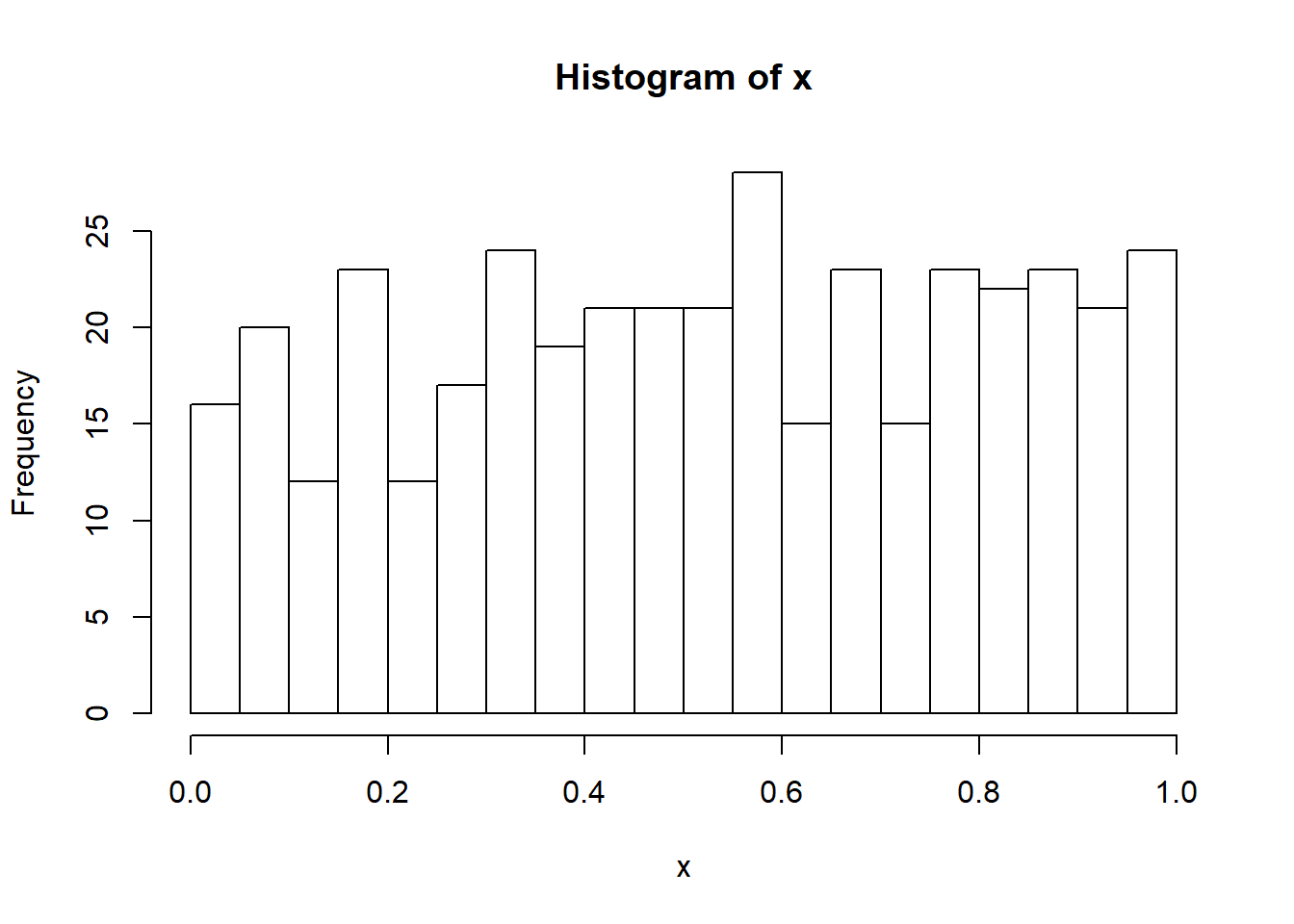# b.
# The categories: [0, 0.1], (0.1, 0.2],...
obs_x <- diff(sapply(seq(0, 1, by = 0.1), function(i) sum(x <= i)))

# c.
# Each category has the same width 0.1 and the postulated distribution is uniform on [0, 1]
# -> the expected category probabilities are each 0.10 (40 obs. per category)
p_x <- rep(0.1, 10)

# d.
# The null hypothesis is that the data was generated by the uniform distribution on [0, 1]
# and the alternative that it was not.
chisq.test(x = obs_x, p = p_x)
##
##  Chi-squared test for given probabilities
##
## data:  obs_x
## X-squared = 7.85, df = 9, p-value = 0.5493
# e.
# The test p-value is 0.5493
# -> no evidence against H0, it is still plausible that the data is from Uniform[0, 1].
# By choosing the categories suitably, it is most likely possible to get the opposite result
# (recall the Type I and II errors). However, this should not be taken advantage of in practice...

1. The data set Titanic contains information on the fate of passengers on the fatal maiden voyage of the ocean liner “Titanic”. We use the data to study whether there is a connection between the sex (Male/Female) of a passenger and surviving from the ship (No/Yes).
1. Extract a marginal table containing only the cross-tabulation of the variables Sex and Survived.
2. Find a suitable way to visualize the data.
3. Which test is appropriate for these data (and why?), $$\chi^2$$ homogeneity test or the $$\chi^2$$ test for independence?
4. Conduct your chosen test on significance level 0.05 and state your conclusions.
# a.
x <- margin.table(Titanic, c(2, 4))

# b.
# Mosaic plot reveals the proportions of survived passengers for both sexes.
# -> proportionally fewer male passengers survived than female. To see whether this effect is statistically
# significant (and not caused just by randomness), we conduct an appropriate test.
mosaicplot(x)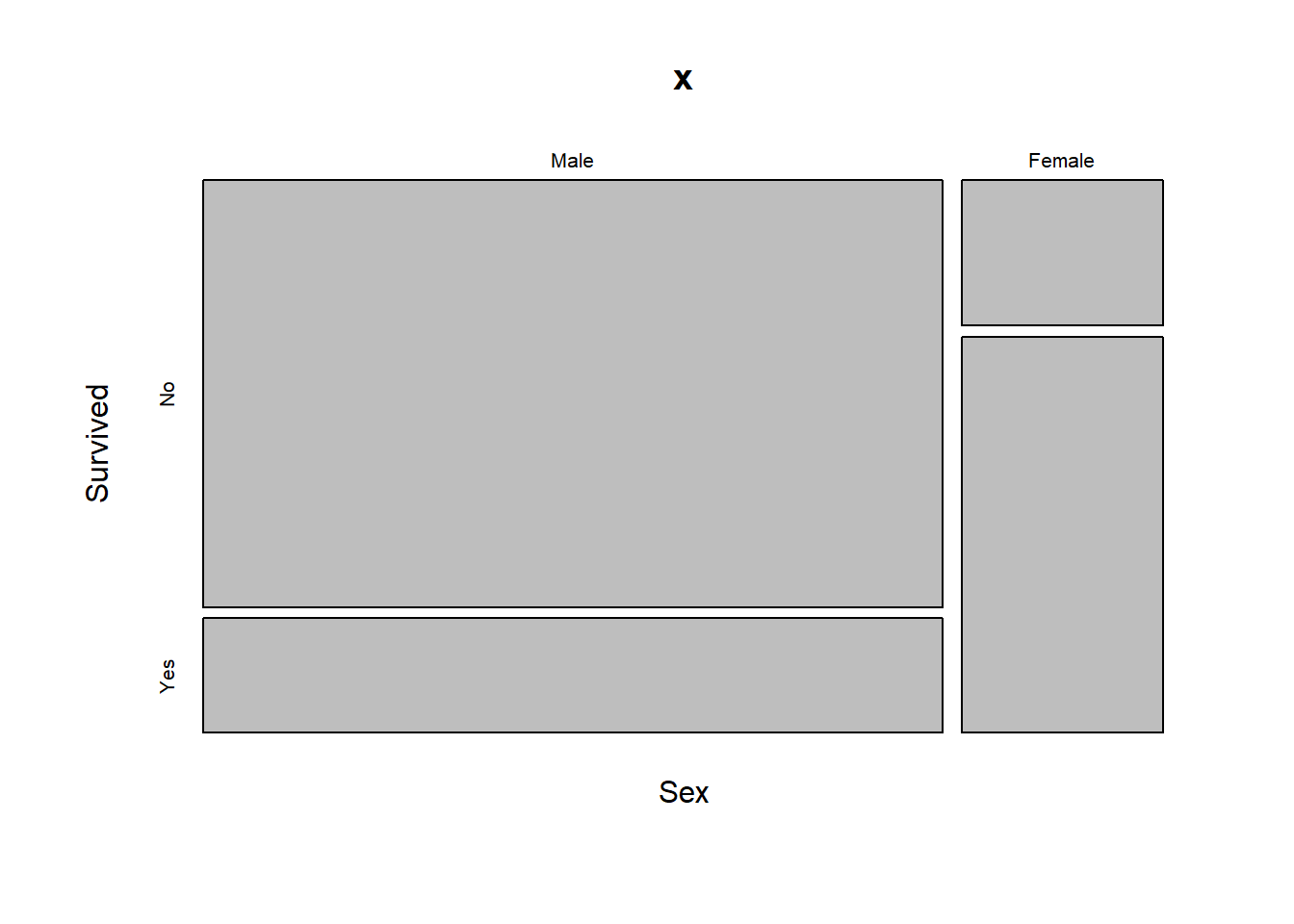# c.
# It seems plausible that there were no quotas on Female/Male passengers on the ship. As such, both factors had
# their margins non-fixed and the correct test is the test for independence.

# d.
chisq.test(x)
##
##  Pearson's Chi-squared test with Yates' continuity correction
##
## data:  x
## X-squared = 454.5, df = 1, p-value < 2.2e-16
# Very low p-value
# -> sex and survival status are not independent -> females had stat. significant higher chance of surviving.

1. (Optional) Choose your favorite non-normal distribution and use simulations to study the Type II error probabilities of the Bowman-Shenton (Jarque-Bera) and Shapiro-Wilk tests of normality for that distribution on different sample sizes (e.g. $$n = 10, 100, 1000, 10000$$). That is, find out the probabilty of falsely concluding that the data comes from a normal distribution when it does not.